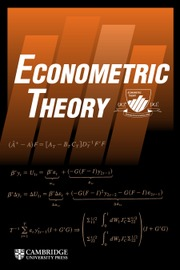Home
Hostname: page-component-5c569c448b-9hjnw Total loading time: 0.928 Render date: 2022-07-01T09:08:51.119Z Has data issue: true Feature Flags: { "shouldUseShareProductTool": true, "shouldUseHypothesis": true, "isUnsiloEnabled": true, "useRatesEcommerce": false, "useNewApi": true } hasContentIssueEconometric Theory

# LOCAL RANK ESTIMATION OF TRANSFORMATION MODELS WITH FUNCTIONAL COEFFICIENTS

Published online by Cambridge University Press:  22 March 2010

## Abstract

This paper considers a nonparametric functional coefficient model with an unknown link function. The model gives flexibility to the standard interaction-variable model by allowing an arbitrary functional form of heterogeneous marginal effects. A local rank estimation procedure is proposed for the functional coefficients along with its asymptotic property.

Type
Brief Report
Information
Econometric Theory , December 2010 , pp. 1807 - 1819

## Access options

Get access to the full version of this content by using one of the access options below. (Log in options will check for institutional or personal access. Content may require purchase if you do not have access.)

## Footnotes

This paper is based on a part of my thesis. I thank Shakeeb Khan for his encouragement and invaluable guidance. I am also grateful to Jinyong Hahn (coeditor), two anonymous referees, Yulia Kotlyarova, Hiro Kasahara, Sung Jae Jun, and seminar participants at various places for helpful comments on the previous version that led to significant improvements. All errors are mine.

## References

Abrevaya, J. (1999a) Computation of the maximum rank correlation estimator. Economics Letters 62, 279285.CrossRefGoogle Scholar
Abrevaya, J. (1999b) Leapfrog estimation of a fixed-effects model with unknown transformation of the dependent variable. Journal of Econometrics 93, 203228.CrossRefGoogle Scholar
Carroll, R.J., Fan, J., Gijbels, I., & Wand, M.P. (1997) Generalized partially linear single-index models. Journal of the American Statistical Association 92, 477489.CrossRefGoogle Scholar
Cavanagh, C. & Sherman, R. (1998) Rank estimation for monotonic index models. Journal of Econometrics 84, 351381.CrossRefGoogle Scholar
Chen, S. (2002) Rank estimation of transformation models. Econometrica 70, 16831697.10.1111/1468-0262.00347CrossRefGoogle Scholar
Cleveland, W.S., Grosse, E., & Shyu, W.M. (1991) Local regression models. In Chambers, J.M. & Hastie, T.J. (eds.), Statistical Models in S, pp. 309376. Chapman and Hall.Google Scholar
Fengler, M.R., Härdle, W.K., & Mammen, E. (2007) A semiparametric factor model for implied volatility surface dynamics. Journal of Financial Econometrics 5, 189218.CrossRefGoogle Scholar
Gelfand, A.E., Kim, H.-J., Sirmans, C.F., & Banerjee, S. (2003) Spatial modeling with spatially varying coefficient processes. Journal of the American Statistical Association 98, 387396.10.1198/016214503000170CrossRefGoogle Scholar
Han, A. (1987) Non-parametric analysis of a generalized regression model. Journal of Econometrics 35, 303316.CrossRefGoogle Scholar
Hastie, T. & Tibshirani, R. (1993) Varying-coefficient models. Journal of the Royal Statistical Society, Series B 55, 757796.Google Scholar
Hoeffding, W. (1948) A class of statistics with asymptotically normal distribution. Annals of Mathematical Statistics 19, 293325.10.1214/aoms/1177730196CrossRefGoogle Scholar
Horowitz, J. (1996) Semiparametric estimation of a regression model with an unknown transformation of the dependent variable. Econometrica 64, 103137.10.2307/2171926CrossRefGoogle Scholar
Horowitz, J. (2001) Nonparametric estimation of a generalized additive model with an unknown link function. Econometrica 69, 499513.CrossRefGoogle Scholar
Kauermann, G., Tutz, G., & Brüerl, J. (2005) The survival of newly founded firms: A case-study into varying-coefficient models. Journal of the Royal Statistical Society Series, A 168, 145158.CrossRefGoogle Scholar
Khan, S. & Tamer, E. (2007) Partial rank estimation of transformation models with general forms of censoring. Journal of Econometrics 136, 251280.CrossRefGoogle Scholar
Klein, R.W. & Sherman, R.P. (2002) Shift restrictions and semiparametric estimation in ordered response models. Econometrica 70, 663691.CrossRefGoogle Scholar
Manski, C. (1985) Semiparametric analysis of discrete response: Asymptotic properties of maximum score estimation. Journal of Econometrics 27, 313334.CrossRefGoogle Scholar
Newey, W.K. & McFadden, D. (1994) Large sample estimation and hypothesis testing. In Engle, R.F. & McFadden, D. (eds.), Handbook of Econometrics, vol. 4, pp. 21112245. Elsevier.Google Scholar
Serfling, R. (1980) Approximation Theorems of Mathematical Statistics. Wiley.CrossRefGoogle Scholar
Sherman, R. (1993) The limiting distribution of the maximum rank correlation estimator. Econometrica 61, 123137.CrossRefGoogle Scholar
Sherman, R. (1994a) Maximal inequalities for degenerate U-processes with application to optimization estimators. Annals of Statistics 22, 439459.CrossRefGoogle Scholar
Sherman, R. (1994b) U-processes in the analysis of generalized semiparametric regression estimator. Econometric Theory 10, 372395.CrossRefGoogle Scholar
Ye, J. & Duan, N. (1997) Nonparametric-consistent estimation for the general transformation model. Annals of Statistics 25, 26822717.CrossRefGoogle Scholar
12
Cited by

# Save article to Kindle

Note you can select to save to either the @free.kindle.com or @kindle.com variations. ‘@free.kindle.com’ emails are free but can only be saved to your device when it is connected to wi-fi. ‘@kindle.com’ emails can be delivered even when you are not connected to wi-fi, but note that service fees apply.

Find out more about the Kindle Personal Document Service.

LOCAL RANK ESTIMATION OF TRANSFORMATION MODELS WITH FUNCTIONAL COEFFICIENTS
Available formats
×

# Save article to Dropbox

To save this article to your Dropbox account, please select one or more formats and confirm that you agree to abide by our usage policies. If this is the first time you used this feature, you will be asked to authorise Cambridge Core to connect with your Dropbox account. Find out more about saving content to Dropbox.

LOCAL RANK ESTIMATION OF TRANSFORMATION MODELS WITH FUNCTIONAL COEFFICIENTS
Available formats
×

# Save article to Google Drive

To save this article to your Google Drive account, please select one or more formats and confirm that you agree to abide by our usage policies. If this is the first time you used this feature, you will be asked to authorise Cambridge Core to connect with your Google Drive account. Find out more about saving content to Google Drive.

LOCAL RANK ESTIMATION OF TRANSFORMATION MODELS WITH FUNCTIONAL COEFFICIENTS
Available formats
×
×